This is one of the 100+ free recipes of the IPython Cookbook, Second Edition, by Cyrille Rossant, a guide to numerical computing and data science in the Jupyter Notebook. The ebook and printed book are available for purchase at Packt Publishing.

▶  Text on GitHub with a CC-BY-NC-ND license
▶  Code on GitHub with a MIT license

Vega is a declarative format for designing static and interactive visualizations. It provides a JSON-based visualization grammar that focuses on the what instead of the how. Vega-Lite is a higher-level specification that is easier to use than Vega, and that compiles directly to Vega.

Altair is a Python library that provides a simple API to define and display Vega-Lite visualizations. It works in the Jupyter Notebook, JupyterLab, and nteract.

Altair is under active development and some details of the API might change in future versions.

## Getting started...

Install Altair with `conda install -c conda-forge altair`.

## How to do it...

1.  Let's import Altair:

```import altair as alt
```

2.  Altair provides several example datasets:

```alt.list_datasets()
```
```['airports',
...
'driving',
'flare',
'flights-10k',
'flights-20k',
'flights-2k',
'flights-3m',
'flights-5k',
'flights-airport',
'gapminder',
...
'wheat',
'world-110m']
```

3.  We load the `flights-5k` dataset:

```df = alt.load_dataset('flights-5k')
```

The `load_dataset()` function returns a pandas `DataFrame`.

```df.head(3)
```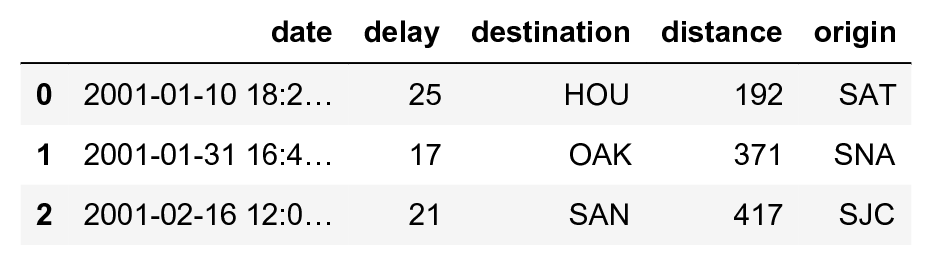This dataset provides the date, origin, destination, flight distance, and delay of many flights.

4.  Let's create a scatter plot showing the delay as a function of the date, with the marker size depending on the flight distance:

```alt.Chart(df).mark_point().encode(
x='date',
y='delay',
size='distance',
)
```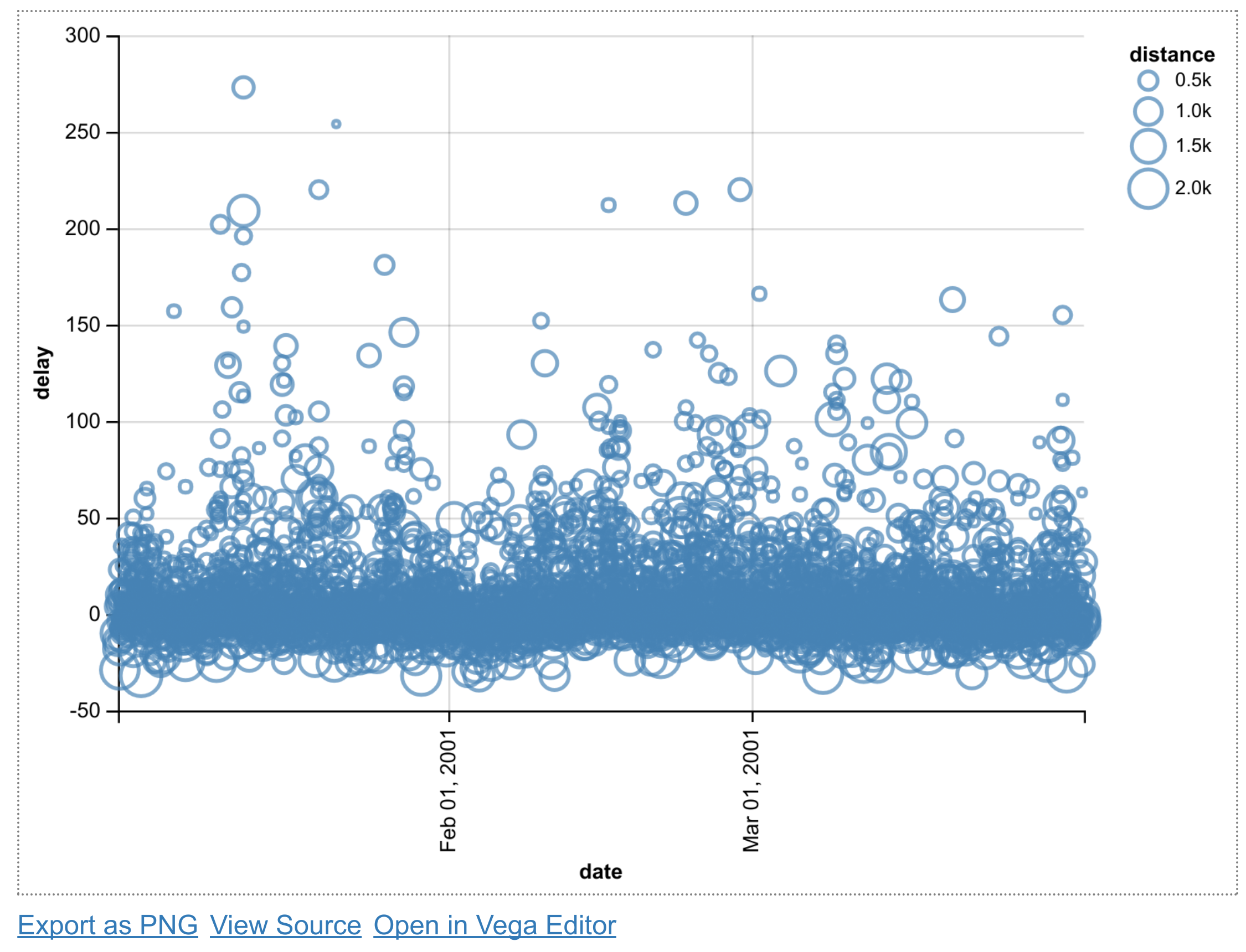The `mark_point()` method specifies that we're creating a scatter plot. The `encode()` function allows us to link parameters of the plot (x and y coordinates, the point size) to specific columns in our DataFrame.

5.  Next, we create a bar plot with the average delay of all flights departing from Los Angeles, as a function of time:

```df_la = df[df['origin'] == 'LAX']

x = alt.X('date', bin=True)
y = 'average(delay)'

alt.Chart(df_la).mark_bar().encode(
x=x,
y=y,
)
```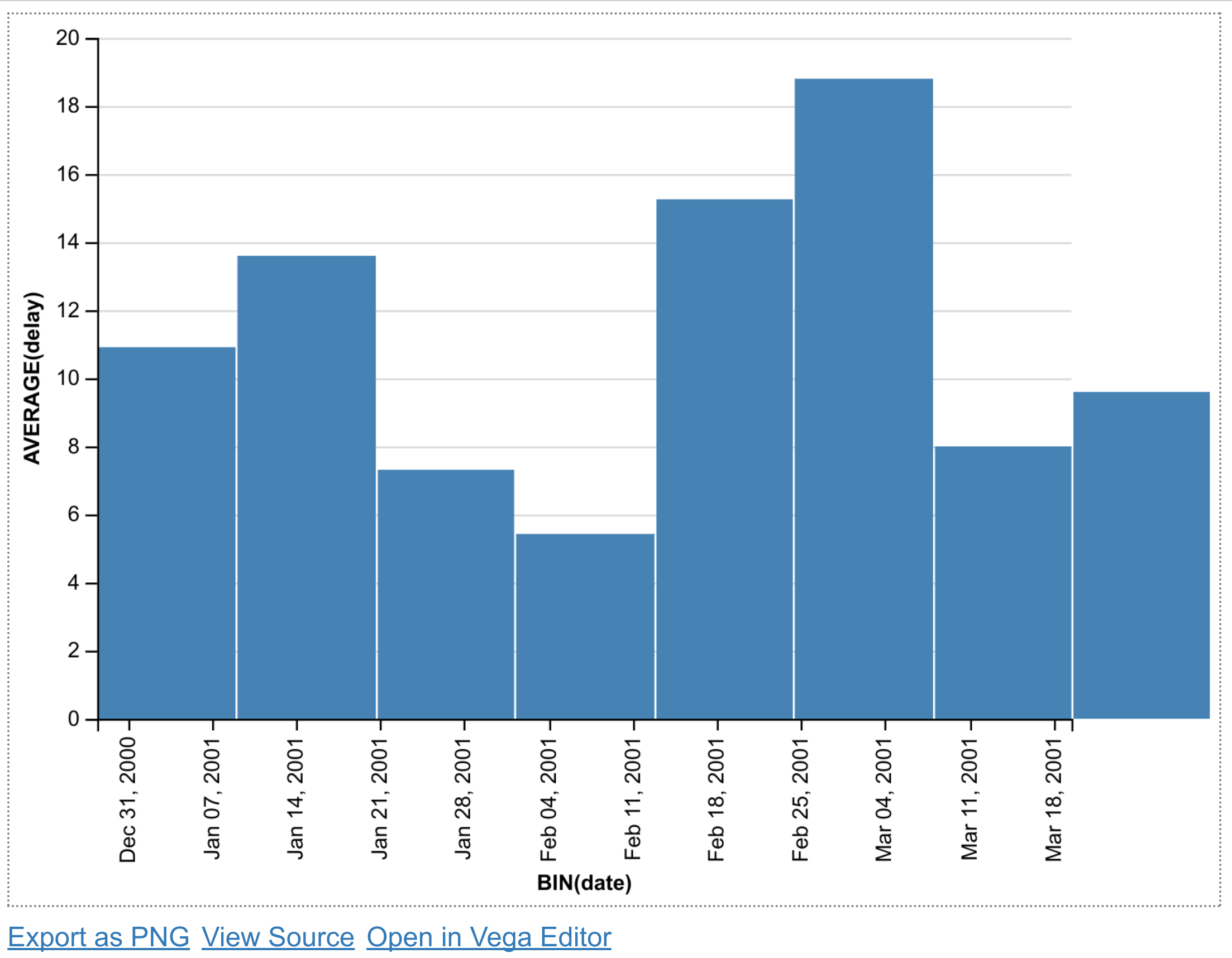We select all flights departing from the `LAX` airport using pandas. For the x coordinate, we use the `alt.X` class to specify that we want a histogram (`bin=True`). For the y coordinate, we specify the average of all delays for every bin.

6.  Now, we create a histogram of the average delay of every origin airport. We use the `sort` option of the `X` class to specify that we want to order the x axis (origin) as a function of the average delay, in descending order:

```sort_delay = alt.SortField(
'delay', op='average', order='descending')

x = alt.X('origin', sort=sort_delay)
y = 'average(delay)'

alt.Chart(df).mark_bar().encode(
x=x,
y=y,
)
```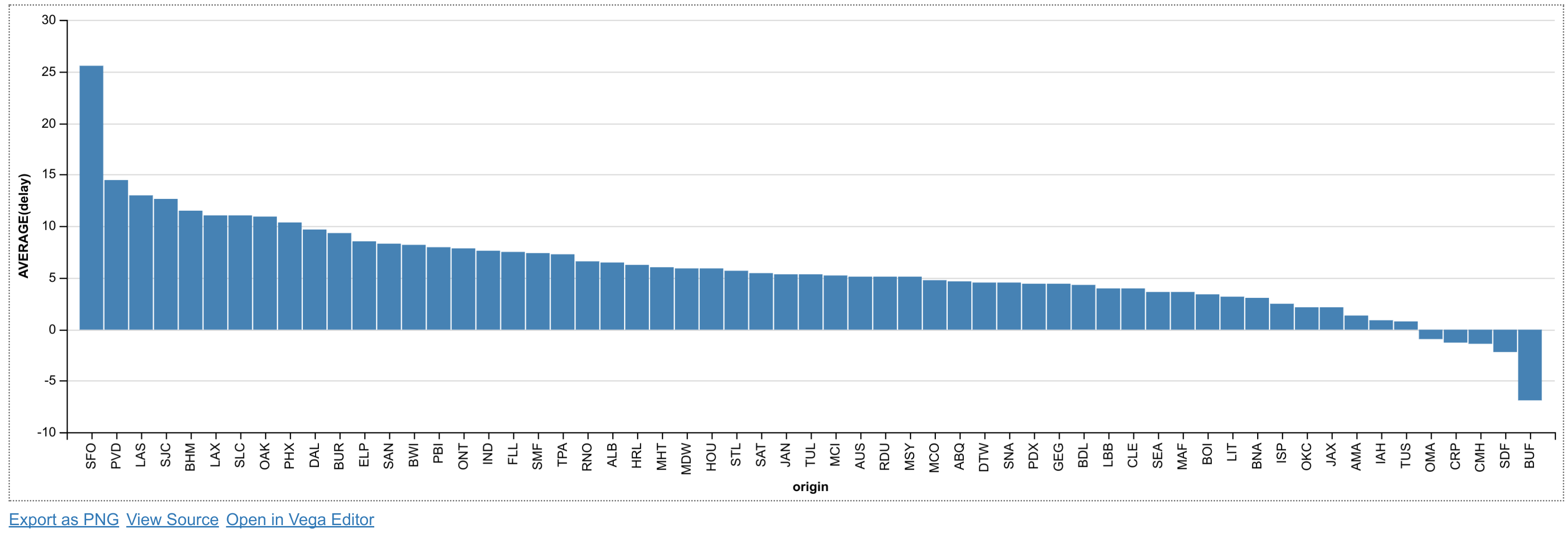## How it works...

Altair provides a Python API to generate a Vega-Lite specification in JSON. The `to_json()` method of an Altair chart can be used to inspect the JSON created by Altair. For example, here is the JSON of the last chart example above:

```{
"\$schema": "https://vega.github.io/schema/vega-lite/v1.2.1.json",
"data": {
"values": [
{
"date": "2001-01-10 18:20:00",
"delay": 25,
"destination": "HOU",
"distance": 192,
"origin": "SAT"
},
...
]
},
"encoding": {
"x": {
"field": "origin",
"sort": {
"field": "delay",
"op": "average",
"order": "descending"
},
"type": "nominal"
},
"y": {
"aggregate": "average",
"field": "delay",
"type": "quantitative"
}
},
"mark": "bar"
}
```

The JSON may contain the data itself, like here, or a URL to a data file. It also defines the encoding channels which link the chart parameters to the data.

In the Jupyter Notebook, Altair leverages the Vega-Lite library to create a Canvas or SVG figure with the requested plot.

## There's more...

Altair and Vega-Lite support much more complex charts, as can be seen in the galleries of these projects.

Vega-Lite supports interactive plots. The following example from the Vega-Lite gallery illustrates linked brushing between subplots, where a rectangular selection can be drawn with the mouse in any subplot: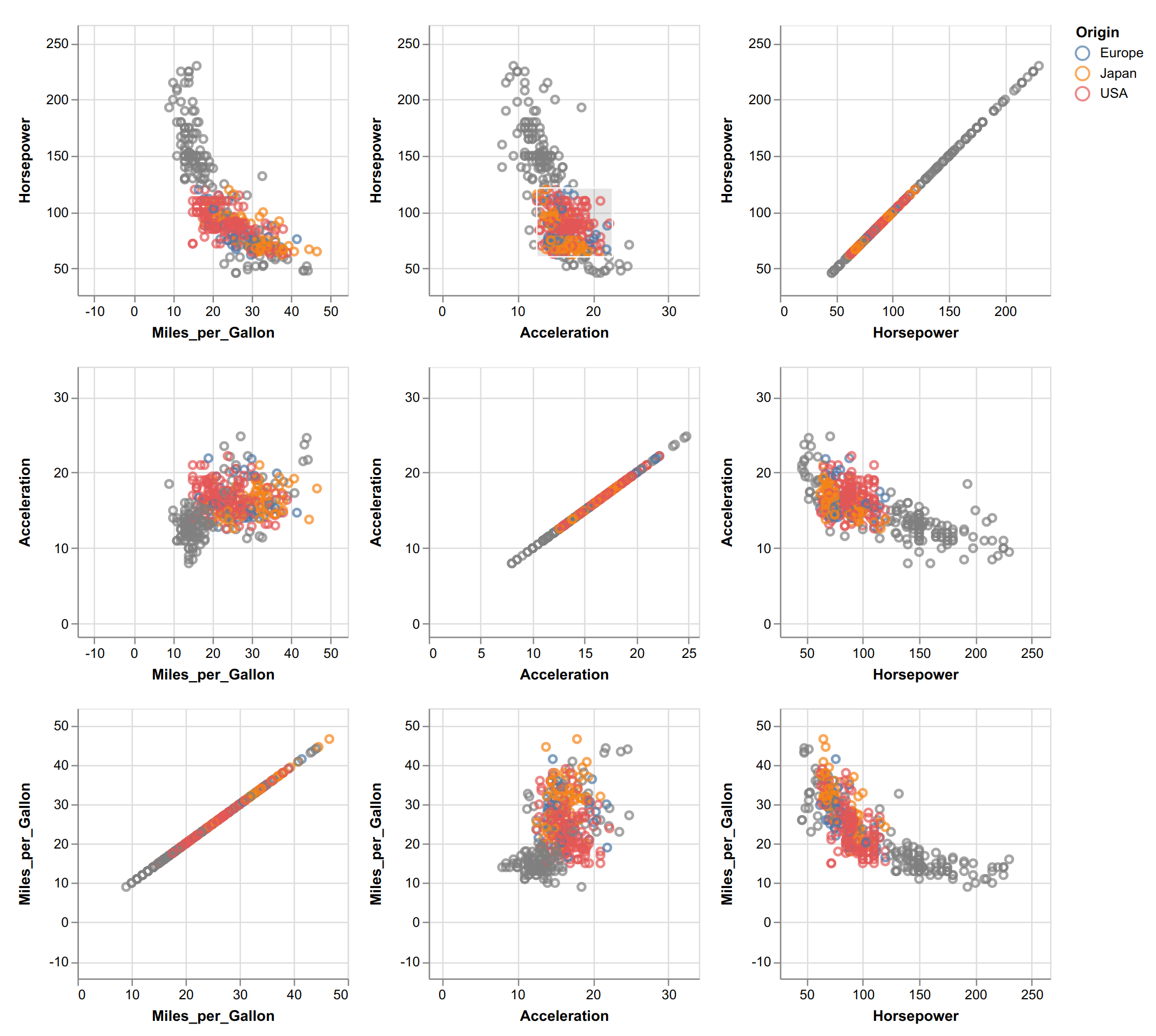There is also an online editor on the Vega-Lite website that can be used to create plots directly in the browser without installing anything.

Here are a few references: